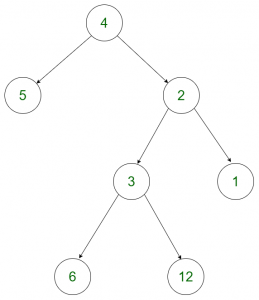# Find the maximum GCD of the siblings of a Binary Tree

Given a 2d-array arr[][] which represents the nodes of a Binary tree, the task is to find the maximum GCD of the siblings of this tree without actually constructing it.

Example:

Input: arr[][] = {{4, 5}, {4, 2}, {2, 3}, {2, 1}, {3, 6}, {3, 12}}
Output: 6
Explanation:For the above tree, the maximum GCD for the sibilings is formed for the nodes 6 and 12 for the children of node 3.

Input: arr[][] = {{5, 4}, {5, 8}, {4, 6}, {4, 9}, {8, 10}, {10, 20}, {10, 30}}
Output: 10

## Recommended: Please try your approach on {IDE} first, before moving on to the solution.

Approach: The idea is to form a vector and store the tree in the form of the vector. After storing the tree in the form of a vector, the following cases occur:

1. If the vector size is 0 or 1, then print 0 as GCD could not be found.
2. For all other cases, since we store the tree in the form of a pair, we consider the first values of two pairs and compare them.
For example, let’s assume there are two pairs in the vector A and B. We check if:

```A.first == B.first
```

If both of them match, then both of them belongs to the same parent. Therefore, we compute the GCD of the second values in the pairs and finally print the maximum of all such GCD’s.

Below is the implementation of the above approach:

## CPP

 `// C++ program to find the maximum ` `// GCD of the siblings of a binary tree ` ` `  `#include ` `using` `namespace` `std; ` ` `  `// Function to find maximum GCD ` `int` `max_gcd(vector >& v) ` `{ ` `    ``// No child or Single child ` `    ``if` `(v.size() == 1 || v.size() == 0) ` `        ``return` `0; ` ` `  `    ``// To get the first pair ` `    ``pair<``int``, ``int``> a = v; ` `    ``pair<``int``, ``int``> b; ` `    ``int` `ans = INT_MIN; ` `    ``for` `(``int` `i = 1; i < v.size(); i++) { ` `        ``b = v[i]; ` ` `  `        ``// If both the pairs belongs to ` `        ``// the same parent ` `        ``if` `(b.first == a.first) ` ` `  `            ``// Update ans with the max ` `            ``// of current gcd and ` `            ``// gcd of both children ` `            ``ans ` `                ``= max(ans, ` `                      ``__gcd(a.second, ` `                            ``b.second)); ` ` `  `        ``// Update previous ` `        ``// for next iteration ` `        ``a = b; ` `    ``} ` `    ``return` `ans; ` `} ` ` `  `// Driver function ` `int` `main() ` `{ ` `    ``vector > v; ` `    ``v.push_back(make_pair(5, 4)); ` `    ``v.push_back(make_pair(5, 8)); ` `    ``v.push_back(make_pair(4, 6)); ` `    ``v.push_back(make_pair(4, 9)); ` `    ``v.push_back(make_pair(8, 10)); ` `    ``v.push_back(make_pair(10, 20)); ` `    ``v.push_back(make_pair(10, 30)); ` ` `  `    ``cout << max_gcd(v); ` `    ``return` `0; ` `} `

## python3

 `# Python3 program to find the maximum ` `# GCD of the siblings of a binary tree ` `from` `math ``import` `gcd ` ` `  `# Function to find maximum GCD ` `def` `max_gcd(v): ` ` `  `    ``# No child or Single child ` `    ``if` `(``len``(v) ``=``=` `1` `or` `len``(v) ``=``=` `0``): ` `        ``return` `0` ` `  `    ``# To get the first pair ` `    ``a ``=` `v[``0``] ` `    ``ans ``=` `-``10``*``*``9` `    ``for` `i ``in` `range``(``1``, ``len``(v)): ` `        ``b ``=` `v[i] ` ` `  `        ``# If both the pairs belongs to ` `        ``# the same parent ` `        ``if` `(b[``0``] ``=``=` `a[``0``]): ` ` `  `            ``# Update ans with the max ` `            ``# of current gcd and ` `            ``# gcd of both children ` `            ``ans ``=` `max``(ans, gcd(a[``1``], b[``1``])) ` ` `  `        ``# Update previous ` `        ``# for next iteration ` `        ``a ``=` `b ` `    ``return` `ans ` ` `  `# Driver function ` `if` `__name__ ``=``=` `'__main__'``: ` `    ``v``=``[] ` `    ``v.append([``5``, ``4``]) ` `    ``v.append([``5``, ``8``]) ` `    ``v.append([``4``, ``6``]) ` `    ``v.append([``4``, ``9``]) ` `    ``v.append([``8``, ``10``]) ` `    ``v.append([``10``, ``20``]) ` `    ``v.append([``10``, ``30``]) ` ` `  `    ``print``(max_gcd(v)) ` ` `  `# This code is contributed by mohit kumar 29     `

Output:

```10
```

Attention reader! Don’t stop learning now. Get hold of all the important DSA concepts with the DSA Self Paced Course at a student-friendly price and become industry ready.

My Personal Notes arrow_drop_upCheck out this Author's contributed articles.

If you like GeeksforGeeks and would like to contribute, you can also write an article using contribute.geeksforgeeks.org or mail your article to contribute@geeksforgeeks.org. See your article appearing on the GeeksforGeeks main page and help other Geeks.

Please Improve this article if you find anything incorrect by clicking on the "Improve Article" button below.

Improved By : mohit kumar 29

Article Tags :
Practice Tags :

Be the First to upvote.

Please write to us at contribute@geeksforgeeks.org to report any issue with the above content.